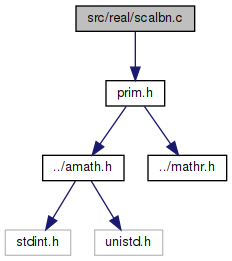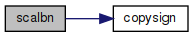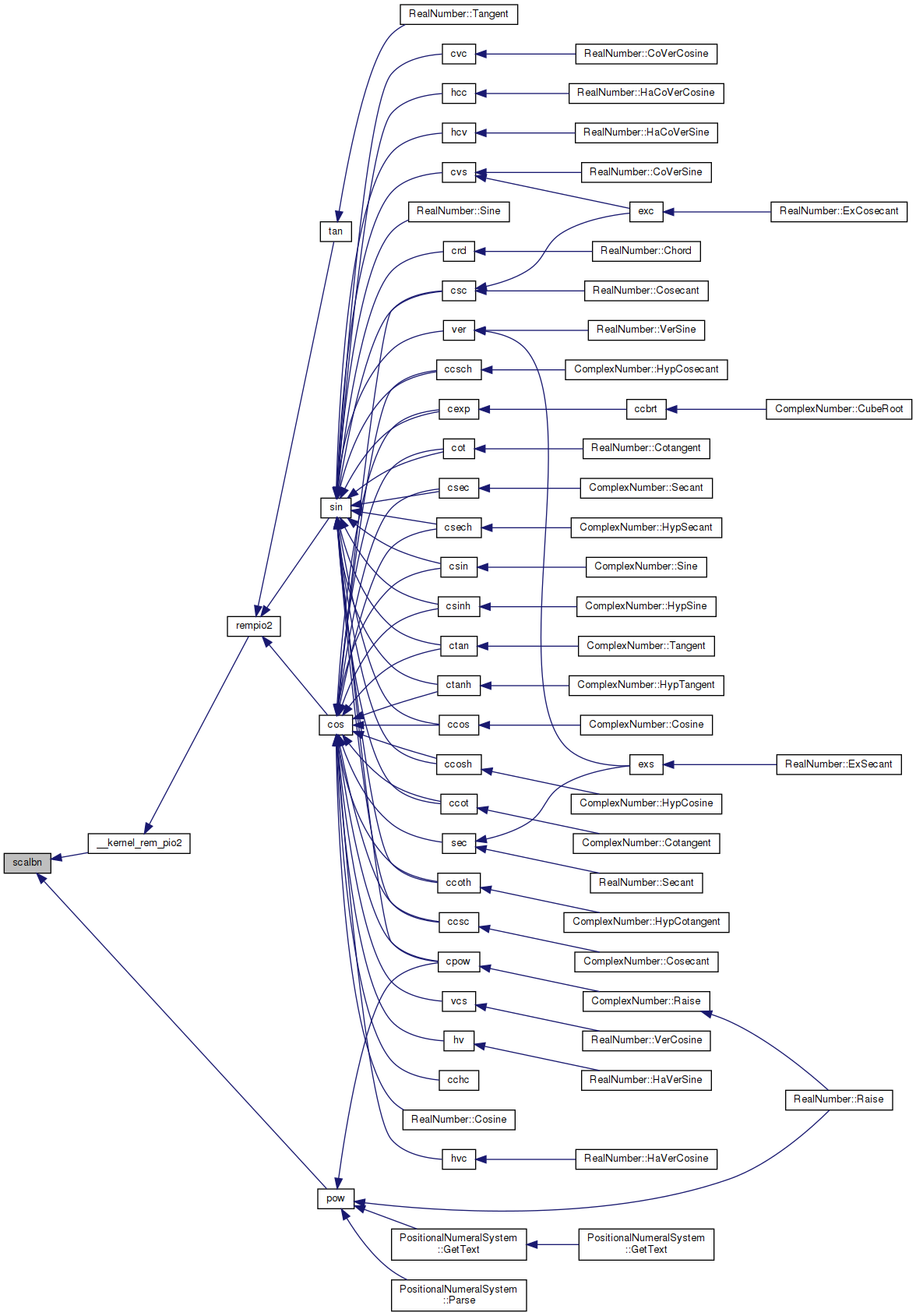amath  1.8.5 Simple command line calculator
scalbn.c File Reference
`#include "prim.h"`
Include dependency graph for scalbn.c:Go to the source code of this file.

## Functions

double scalbn (double x, int32_t n)

## Variables

static const double two54 = 1.80143985094819840000e+16

static const double twom54 = 5.55111512312578270212e-17

static const double huge = 1.0e+300

static const double tiny = 1.0e-300

## ◆ scalbn()

 double scalbn ( double x, int32_t n )

Definition at line 56 of file scalbn.c.

References copysign(), huge, tiny, two54, and twom54.

Referenced by __kernel_rem_pio2(), and pow().

57 {
58  int32_t k, hx, lx;
59  EXTRACT_WORDS(hx, lx, x);
60  k = (hx & 0x7ff00000) >> 20; /* extract exponent */
61  if (k == 0)
62  { /* 0 or subnormal x */
63  if ((lx | (hx & 0x7fffffff)) == 0)
64  return x; /* +-0 */
65  x *= two54;
66  GET_HIGH_WORD(hx, x);
67  k = ((hx & 0x7ff00000) >> 20) - 54;
68  if (n < -50000)
69  return tiny * x; /*underflow*/
70  }
71  if (k == 0x7ff)
72  return x + x; /* NaN or Inf */
73  k = k + n;
74  if (k > 0x7fe)
75  return huge * copysign(huge, x); /* overflow */
76  if (k > 0) /* normal result */
77  {
78  SET_HIGH_WORD(x, (hx & 0x800fffff) | (k << 20));
79  return x;
80  }
81  if (k <= -54)
82  {
83  if (n > 50000) /* in case integer overflow in n+k */
84  return huge * copysign(huge, x); /*overflow*/
85  else
86  return tiny * copysign(tiny, x); /*underflow*/
87  }
88  k += 54; /* subnormal result */
89  SET_HIGH_WORD(x, (hx & 0x800fffff) | (k << 20));
90  return x * twom54;
91 }
#define GET_HIGH_WORD(i, d)
Get the more significant 32 bit int from a double.
Definition: prim.h:167
static const double two54
Definition: scalbn.c:51
static const double tiny
Definition: scalbn.c:54
#define EXTRACT_WORDS(ix0, ix1, d)
Get two 32 bit ints from a double.
Definition: prim.h:156
double copysign(double x, double y)
Returns a value with the magnitude of x and with the sign bit of y.
Definition: csign.c:47
static const double huge
Definition: scalbn.c:53
#define SET_HIGH_WORD(d, v)
Set the more significant 32 bits of a double from an int.
Definition: prim.h:198
static const double twom54
Definition: scalbn.c:52
Here is the call graph for this function:Here is the caller graph for this function:## ◆ huge

 const double huge = 1.0e+300
static

Definition at line 53 of file scalbn.c.

Referenced by scalbn().

## ◆ tiny

 const double tiny = 1.0e-300
static

Definition at line 54 of file scalbn.c.

Referenced by scalbn().

## ◆ two54

 const double two54 = 1.80143985094819840000e+16
static

Definition at line 51 of file scalbn.c.

Referenced by scalbn().

## ◆ twom54

 const double twom54 = 5.55111512312578270212e-17
static

Definition at line 52 of file scalbn.c.

Referenced by scalbn().